# Solve system of linear equations by substitution method onlineThe most simple method to solve system of linear algebraic equations (SLE) is the substitution method. Consider it more detailed, suppose we have SLE of the form: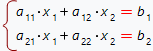We need to solve it, i.e. to find such the values of the variables x1, x2, which convert the initial SLE to the correct identity. The substitution method consists of the following steps:

1. Solve first equation of the SLE with respect to the x1: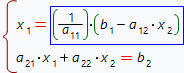2. Substitute obtained identity for x1 variable into the second equation of the SLE: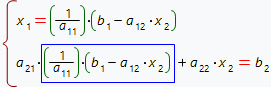3. Simplify second equation of the SLE: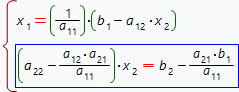4. Solve second equation of the SLE with respect to the x2: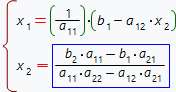5. Substitute received expression for x2 into the first equation of SLE: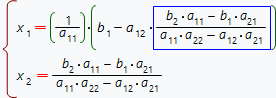6. Simplify first equation of the SLE: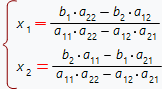This online calculator solves SLE by the substitution method with step by step solution free of charge. The SLE's coefficients can be the numbers, fractions or parameters. To use the calculator, one need to input the SLE in its natural form and choose the variables to solve.

Solve slae by substitution method
,,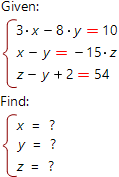System of linear algebraic equations you want to solve: